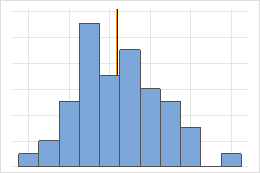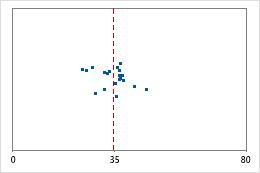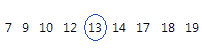# Interpret the results for Column Statistics and Row Statistics

Find definitions and interpretation guidance for every statistic that is provided with column statistics and row statistics.

## Sum

The sum is the total of all the data values. The sum is also used in statistical calculations, such as the mean and standard deviation.

## Mean

The mean is the average of the data, which is the sum of all the observations divided by the number of observations.

For example, the wait times (in minutes) of five customers in a bank are: 3, 2, 4, 1, and 2. The mean waiting time is calculated as follows: On average, a customer waits 2.4 minutes for service at the bank.

### Interpretation

Use the mean to describe the sample with a single value that represents the center of the data. Many statistical analyses use the mean as a standard measure of the center of the distribution of the data.

The median and the mean both measure central tendency. But unusual values, called outliers, can affect the median less than they affect the mean. If your data are symmetric, the mean and median are similar.For the symmetric distribution, the mean (blue line) and median (orange line) are so similar that you can't easily see both lines. But the non-symmetric distribution is skewed to the right.

## StDev

The standard deviation is the most common measure of dispersion, or how spread out the data are about the mean. The symbol σ (sigma) is often used to represent the standard deviation of a population, while s is used to represent the standard deviation of a sample. Variation that is random or natural to a process is often referred to as noise.

Because the standard deviation is in the same units as the data, it is usually easier to interpret than the variance.

### Interpretation

Use the standard deviation to determine how spread out the data are from the mean. A higher standard deviation value indicates greater spread in the data. A good rule of thumb for a normal distribution is that approximately 68% of the values fall within one standard deviation of the mean, 95% of the values fall within two standard deviations, and 99.7% of the values fall within three standard deviations.

The standard deviation can also be used to establish a benchmark for estimating the overall variation of a process.Hospital discharge timesAdministrators track the discharge time for patients who are treated in the emergency departments of two hospitals. Although the average discharge times are about the same (35 minutes), the standard deviations are significantly different. The standard deviation for hospital 1 is about 6. On average, a patient's discharge time deviates from the mean (dashed line) by about 6 minutes. The standard deviation for hospital 2 is about 20. On average, a patient's discharge time deviates from the mean (dashed line) by about 20 minutes.

## Minimum

The minimum is the smallest data value.

In these data, the minimum is 7.

 13 17 18 19 12 10 7 9 14

### Interpretation

Use the minimum to identify a possible outlier or a data-entry error. One of the simplest ways to assess the spread of your data is to compare the minimum and maximum. If the minimum value is very low, even when you consider the center, the spread, and the shape of the data, investigate the cause of the extreme value.

## Maximum

The maximum is the largest data value.

In these data, the maximum is 19.

 13 17 18 19 12 10 7 9 14

### Interpretation

Use the maximum to identify a possible outlier or a data-entry error. One of the simplest ways to assess the spread of your data is to compare the minimum and maximum. If the maximum value is very high, even when you consider the center, the spread, and the shape of the data, investigate the cause of the extreme value.

## Range

The range is the difference between the largest and smallest data values in the sample. The range represents the interval that contains all the data values.

### Interpretation

Use the range to understand the amount of dispersion in the data. A large range value indicates greater dispersion in the data. A small range value indicates that there is less dispersion in the data. Because the range is calculated using only two data values, it is more useful with small data sets.

## Median

The median is the midpoint of the data set. This midpoint value is the point at which half the observations are above the value and half the observations are below the value. The median is determined by ranking the observations and finding the observation that are at the number [N + 1] / 2 in the ranked order. If the number of observations are even, then the median is the average value of the observations that are ranked at numbers N / 2 and [N / 2] + 1.For this ordered data, the median is 13. That is, half the values are less than or equal to 13, and half the values are greater than or equal to 13. If you add another observation equal to 20, the median is 13.5, which is the average between 5th observation (13) and the 6th observation (14).

### Interpretation

The median and the mean both measure central tendency. But unusual values, called outliers, can affect the median less than they affect the mean. If your data are symmetric, the mean and median are similar.For the symmetric distribution, the mean (blue line) and median (orange line) are so similar that you can't easily see both lines. But the non-symmetric distribution is skewed to the right.

## Sum of Squares

The uncorrected sum of squares are calculated by squaring each value in the column, and calculates the sum of those squared values. For example, if the column contains x1, x2, ... , xn, then sum of squares calculates (x12 + x22 + ... + xn2). Unlike the corrected sum of squares, the uncorrected sum of squares includes error. The data values are squared without first subtracting the mean.

## Total Count

The total number of observations in the column. Use to represent the sum of N missing and N nonmissing.

In this example, there are 141 valid observations and 8 missing values. The total count is 149.
Total count N N*
149 141 8

## N

The number of non-missing values in the sample.

In this example, there are 141 recorded observations.
Total count N N*
149 141 8

## N*

The number of missing values in the sample. The number of missing values refers to cells that contain the missing value symbol *.

In this example, 8 errors occurred during data collection and are recorded as missing values.
Total count N N*
149 141 8
By using this site you agree to the use of cookies for analytics and personalized content.  Read our policy